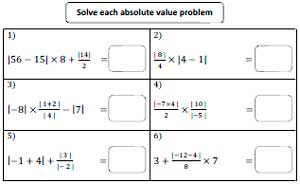1. Worksheets>
2. Math>
3. Pre-Algebra>
4. Absolute Value

# Absolute Value Worksheets

Our printable absolute value worksheets meticulously designed for 6th grade and 7th grade students include exercises like finding the absolute value of positive and negative integers, performing simple addition, subtraction, multiplication and division involving the absolute value of real numbers and more. Begin your practice with our free worksheets.

## Basic Worksheets

Basic Absolute Value Worksheets

Find the absolute value of the integers. This stock of pdf worksheets featuring integers within 100 offers the much-needed practice for 6th grade students.

Simple Absolute Value 2

Simple Absolute Value 3

Find the Absolute Value: Challenging

These printable handouts include questions on finding the absolute value of numbers with or without a negative sign.

Challenging Absolute Value 2

Challenging Absolute Value 3

Add two absolute value of integers.

Subtracting Absolute Value Worksheets

Subtract two absolute value of integers.

Absolute Value Subtraction 2

Combo

It includes both addition and subtraction of absolute value of integers.

## Multiplication and Division

Multiplying Absolute Value Worksheets

Multiply two absolute value of integers.

Absolute Value Multiplication 2

Dividing Absolute Value Worksheets

Divide two absolute value of integers.

Absolute Value Division 2

Combo

It includes both multiplication and division of absolute value of integers.

Multiply or Divide 2

## Evaluate Absolute Value

With this set of printer-friendly worksheets, students are supposed to perform addition or subtraction or both the operations together on expressions involving the absolute value of numbers.

Evaluate the Value 2

Evaluate the Value 3

Multiplication and Division

This bunch of 7th grade worksheets tasks children to first evaluate the absolute value expressions and then multiply, divide and simplify them.

Multiplication and Division 2

Multiplication and Division 3

Mixed Review

Our mixed review pdf worksheets provide ultimate practice in performing all the four arithmetic operations on absolute value expressions. Use PEMDAS to solve the absolute value problems.Absolute Value Worksheet 2

Absolute Value Worksheet 3

Related Worksheets